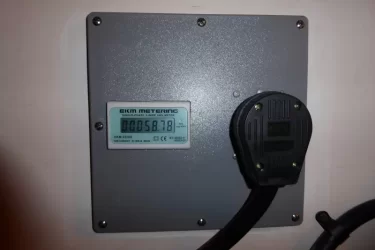# Is 110MPGe possible with the plug-in Clarity?

Discussion in 'Clarity' started by bpratt, Feb 15, 2018.

1. I purchased my Clarity in December and used the 110 volt charger for a few weeks. When I switched to a 240 volt charger, I also installed a KWH meter on the line to the plug.Since then I have been keeping track of my daily mileage and my KWH used. I have never used all the EV range and the engine has never started.
I use an excel spreadsheet to calculate miles/KWH and MPGe the car has gotten. I live in Utah and our current gas price is about \$2.50 per gallon, my current KWH cost is 8.6 cents per KWH. In order to get 110 MPGe, I would have to average 3.784 mile per KWH.
I know its winter and cold and when I run the car the electric heater takes a lot of power, but my lowest miles per KWH has been 2.24 and the highest 3.03. The average is 2.64 which is equal to 76.74 MPGe.
My power cost is very low compared to many places in the country, so I think I should be doing better than I am.

I use the following formula to calculate MPGe:
(cost of gas)/(cost per KWH) * (miles driven)/(KWH used to drive those miles)=MPGe

2. MPGe is officially calculated as (miles driven)/(kwh used from the wall) * 33.4 kwh/gallon. The kwh units cancel out and you are left with MPGe. You are getting 75 to 101 MPGe based on your 2.24 to 3.03 number.

I imagine summer you will exceed 110 MPGe or whatever.

TomW, Michael L. and Kendalf like this.
3. I've been getting about 150 MPGe.50-75 degree temps in winter sure is nice for efficiency!

It seems that we can factor in slightly higher than calculated MPGe results due to energy loses while charging. So if your wall meter measures 10kWh consumed the car's battery didn't actually get all that charge. I've heard about 10% loss with level 2 charging.

4. I am curious how often the Clarity will run engine maintenance modes, if you haven't used any gas yet, you might get to find out. My hunch is 4 to 6 weeks it will force the engine to run for a while, but curious if the car will say anything, or if the engine will just start on you.

5. So, where did you get the 33.4 kwh/gallon figure? If I use that number, a gallon of gas would cost me \$2.87 instead of the \$2.50 that it actually costs in Utah.

6. https://en.m.wikipedia.org/wiki/Miles_per_gallon_gasoline_equivalent

Looks like it's actually 33.7 kWh/gallon. It's a standardized value that is the estimate for how many kWh of electricity is equivalent to one gallon of gasoline. It does not factor local gas or electricity costs, else there would be no way to compare MPGe between any two vehicles due to the huge variance in gas and electricity prices.

7. 33.7 kwh (typo earlier, meant 33.7 kwh per gallon) is the equivalent thermal energy contained in 1 gallon of gasoline. MPGe is a measure of energy used to run an electric car vs a gas car. A car getting 100 mpge is using 1/4 the energy as a gas car that gets 25 mpg, most of the difference is lost as heat out the exhaust pipe.

Michael L. likes this.
8. OK, that makes more sense that what I was trying to do. If a gallon of gas costs \$2.50, I wanted to know how far my car would go on \$2.50 of electricity. I incorrectly thought that is what was meant by MPGe. Thanks for the info.

9. Exactly. The cost for a gas gallon equivalent of electricity will vary from location to location. Some have commented how their very high electricity rates (peak hours) don't save them much over gasoline. For me, electricity is cheap and gasoline is relatively expensive. I drive a lot of miles around town with ample time to charge in between short trips. Thus, a plug in hybrid is perfect for me.

Tony Vo likes this.
10. I use the slightly lower 32.78 kWh/gal figure for E10 gasoline, since it is the most widely sold fuel in this area.